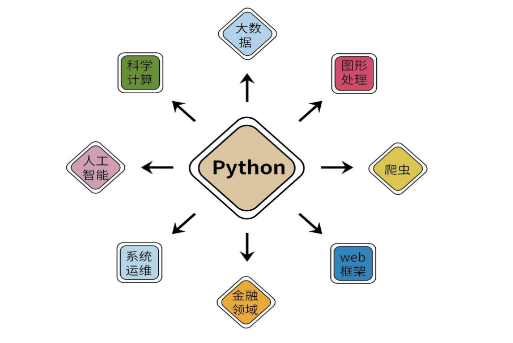# 技术分享

#### 9个技巧使你的Python代码更Python！## 01 product()

### 使用 `product()` 函数避免嵌套的Python循环

``list_a = [1, 2020, 70]list_b = [2, 4, 7, 2000]list_c = [3, 70, 7]for a in list_a:    for b in list_b:        for c in list_c:            if a + b + c == 2077:                print(a, b, c)# 70 2000 7``

``from itertools import productlist_a = [1, 2020, 70]list_b = [2, 4, 7, 2000]list_c = [3, 70, 7]for a, b, c in product(list_a, list_b, list_c):    if a + b + c == 2077:        print(a, b, c)# 70 2000 7``

## 02 海象操作符

### 赋值表达式的一个可爱技巧

``author = "云朵君"print(author)# 云朵君``

``print(author="云朵君")# TypeError: 'author' is an invalid keyword argument for print()``

``print(author:="云朵君")# 云朵君``

## 03 三元条件运算符

### 用一行写一个简单的`If-Else`结构

``min = a if a < b else b``

``if a<b:  min = aelse:  min = b``

## 04 Lambda函数

### 使用Lambda函数来定义简单函数

``def fib(x):    if x<=1:        return x    else:        return fib(x-1) + fib(x-2)``

## 05 列表推导式

### 以Pythonic方式获得一个列表

``Genius = ["云朵君", "小猴子", "数据STUDIO", "机器学习研习院"]L1 = [name if name.startswith('云') else 'Not Genius' for name in Genius]print(L1)# ["云朵君", "Not Genius", "Not Genius", "Not Genius"]``

## 06 高阶函数

### 利用Python中的高阶函数

Python有一些内置的高阶函数，给我们编写一些常见的逻辑提供了便利。

``names = ['yAnG', 'MASk', 'thoMas', 'LISA']names = map(str.capitalize, names)print(list(names))# ['Yang', 'Mask', 'Thomas', 'Lisa']``

``from functools import reducecity = ['L', 'o', 'n', 'd', 'o', 'n', 2, , 2, ]city_to_str = reduce(lambda x, y: str(x) + str(y), city)print(city_to_str)# London2020``

## 07 联合运算符

### 合并字典的简单方法

``article_author = {'数据STUDIO': '云朵君',                   '机器学习研习院': '小猴子'}author_cities = {'云朵君': '江苏',                  '小猴子': '成都'}info = article_author|author_citiesprint(info)#{'数据STUDIO': '云朵君',   '机器学习研习院': '小猴子',   '云朵君': '江苏',   '小猴子': '成都'}``

## 08 F-string

### F-string字符串格式化技术

``pi = 3.1415926print(f'Pi is approximately equal to {pi:.2f}')# Pi is approximately equal to 3.14id = 1  # need to print a 3-digit numberprint(f"The id is {id:03d}")# The id is 001N = 1000000000  # need to add separatorprint(f'His networth is \${N:,d}')# His networth is \$1,000,000,000``

“Simple is better than complex.” — The Zen of Python

``from datetime import datetimeprint(f"Today is {datetime.today()}")# Today is 2022-12-31 18:18:18.666666``

## 9 星号*

### 使用星号来解包迭代变量和解构赋值

``A = [1, 2, 3]B = (4, 5, 6)C = {7, 8, 9}L = [*A, *B, *C]print(L)# [1, 2, 3, 4, 5, 6, 8, 9, 7]``

``a, *mid, b = [1, 2, 3, 4, 5, 6]print(a, mid, b)# 1 [2, 3, 4, 5] 6``